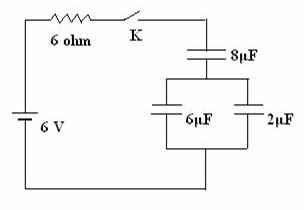## Pages

`“Life is like riding a bicycle.  To keep your balance you must keep moving.”–Albert Einstein`

## Saturday, May 15, 2010

### AP Physics B & C - Multiple Choice Practice Questions on Electric Circuits Containing Resistors and Capacitors

Free response as well as multiple choice questions on direct current circuits containing resistors and capacitors are popular among question setters. Questions involving steady state are meant for AP physics B as well as AP physics C aspirants, where as questions involving RC transients are meant exclusively for AP physics C aspirants.

You will find questions in this section posted earlier on this site by performing a search using the search box on this page or by clicking on the label ‘direct current circuit’ below this page. Today we will discuss a few more practice questions in this section.

The following questions are meant for AP physics B as well as AP physics C aspirants:(1) In the circuit shown in the adjoining figure the capacitors are fully charged. What is the potential difference across the 2 μF capacitor?

(a) 0.5 V

(b) 1 V

(c) 2 V

(d) 3 V

(e) 4 V

The parallel combination of the 6 μF and 2 μF capacitors is equivalent to a single 8 μF capacitor. Therefore, the 6 V battery is (in effect) connected in series with two 8 μF capacitors so that there will be a potential difference of 3 V across each 8 μF capacitor. Since the parallel combination of the 6 μF and 2 μF capacitors makes the lower 8 μF capacitor, the potential difference across the 2 μF capacitor is 3 V.

[The potential difference across the 6 μF capacitor also is 3 V].

(2) In the circuit shown in question no.1 what is the charge on the lower plate of the 6 μF capacitor under steady state?

(a) 48 μC

(b) + 18 μC

(c) – 18 μC

(d) + 12μC

(e) – 12 μC

The lower plate of the 6 μF capacitor is connected to the negative terminal of the battery. The charge on the lower plate is therefore negative. As shown above (while answering question no.1), the potential difference across the 6 μF capacitor is 3 V. Therefore, the charge on the lower plate of the 6 μF capacitor is – (6 μF×3 V) = 18 μC [Remember Q = CV].

(3) In the circuit shown in question no.1 the battery has negligible internal resistance. What is the current through the 6 Ω resistor under steady state?

(a) 0.05 A

(b) 0.2 A

(c) 0.5 A

(d) 1 A

(e) zero

Under steady state the capacitors are fully charged and there is no charging current. The current through the 6 Ω resistor is therefore zero.(4) The circuit shown in the adjoining figure is almost the same as that shown in question no.1, but a key K is included. The internal resistance of the battery is negligible. Suppose the key K is open and the capacitors are initially uncharged. What is the current through the 6 Ω resistor at the moment the key K is closed (and the capacitors begin to get charged)?

(a) 0.05 A

(b) 0.5 A

(c) 1 A

(d) 2 A

(e) zero

At the moment the key is closed, there are no charges on the capacitors to oppose the flow of charges delivered by the battery. The capacitors therefore allow the passage of the transients with ease and the only opposition to the flow of the charges in the circuit is that offered by the resistor. The initial current through the 6 Ω resistor is therefore 6 V/6 Ω = 1 A.

The following questions are for AP Physics C aspirants:

(5) Refer to the circuit shown in question no. 4. Initially the key K is open and the capacitors are uncharged. After closing the key, what time must be elapsed for the current through the 6 Ω resistor to become 1/e ampere (where ‘e’ is the base of natural logarithms)?

(a) 24 μs

(b) 48 μs

(c) 96 μs

(d) 0.01 s

(e) 0.1 s

The initial charging current I0 through the 6 Ω resistor is given by

I0 = V/R = 6 V/6 Ω = 1 A.

The time constant of an RC circuit is the time required for the charging current to reduce from its initial value I0 to (1/e)I0. If you remember this fact, you can immediately write down the answer as RC since I0 = 1 A in the present case. So the answer is 6 Ω×4 μF = 24 μs.

[Usually the time constant of an RC circuit is defined as the time required for the charge on the capacitor (or voltage across the capacitor) to grow from zero to (1– 1/e) times the final steady value. The growth of charge Q is exponential and is given by

Q = Q0 (1 – e–t/RC) where Q0 is the final steady charge.

The charging current I at time t after closing the key K is given by

I = dQ/dt = (Q0/RC)e–t/RC = (CV/RC)e–t/RC = (V/R)e–t/RC = I0e–t/RC.

Therefore, I = (1/e)I0 when t = RC](6) In the circuit shown in the adjoining figure the capacitor C is initially charged to have a potential difference V0 volt across it. The key K is initially open. When the key K is closed (at zero time), the potential difference across the capacitor becomes 0.5 V0 volt after a time t . What is the value of t ?

(a) 2 RC

(b) 0.5RC

(c) RC/ln2

(d) RC ln2

(e) RC

The potential difference V after time t is given by

V = V0 e–t/RC where ‘e’ is the base of natural logarithms.

Therefore we have 0.5 V0 = V0 e–t’/RC.

Or, et’/RC = 1/0.5 = 2 from which t/RC = ln2

This gives t’ = RC ln2.